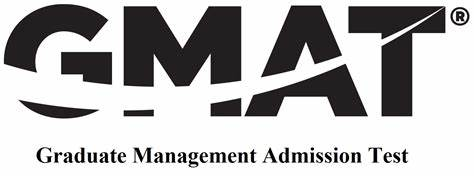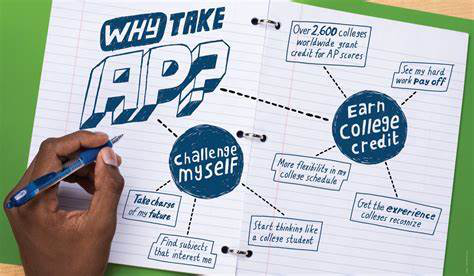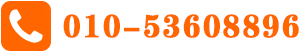还分不清楚吗？数学与附加数学究竟怎么学？【GCSE阶段】

GCSE       时间：2022-01-07 18:35

那我可以看一下考纲里面究竟是怎么规定的：

0580 基础数学
Number，algebra and graphics， coordinate geometry， Maturation Trigeometry, vector and transformations. Probability, statistics.

0606 附加数学
Function, quadratic function, equation, inequity and graphs. factor of polynomial. Equations. Exponetial functions. Straight line graphic. Circular measure. trigonometry. permutations and combinations, series, vectors in two dimensions, differentiation and integration.

那为什么英国的初中和高一的学生还有很多人会选择附加数学这门课程？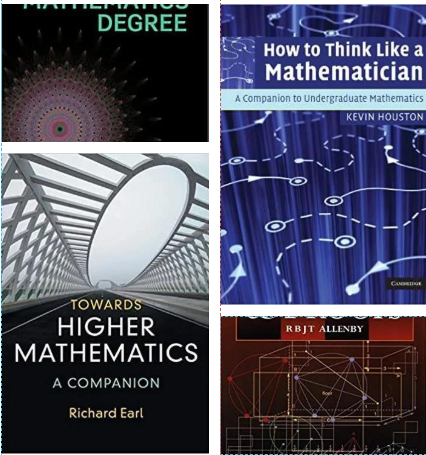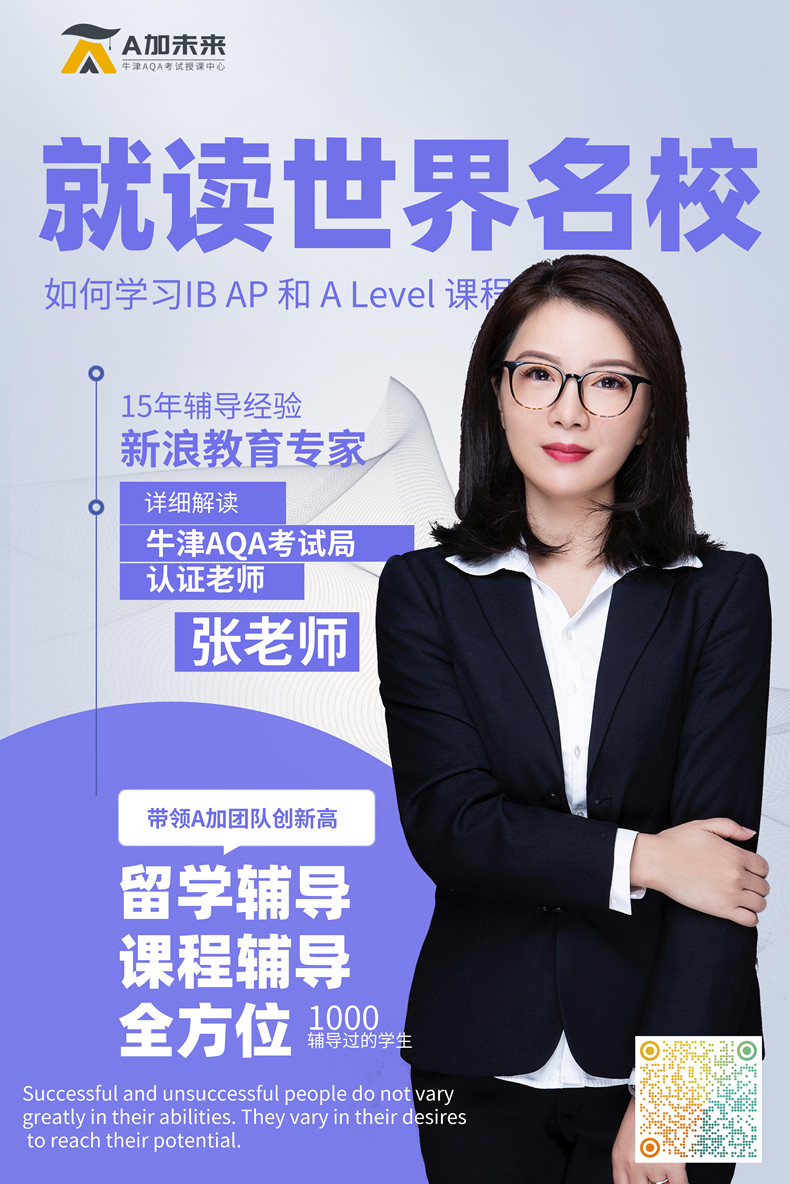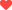喜欢 

最新文章 More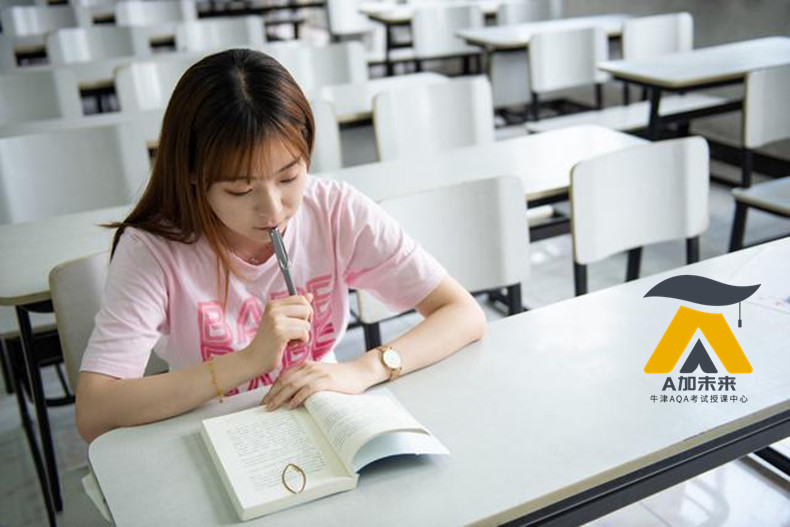相关推荐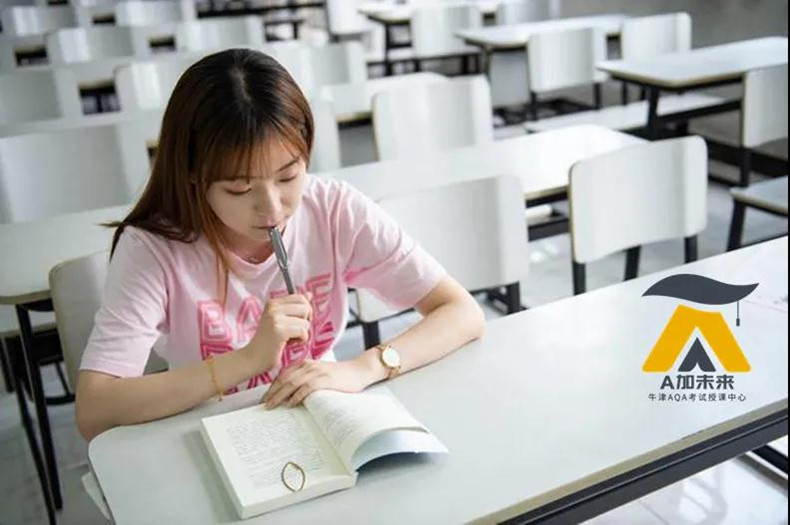猜你喜欢 More